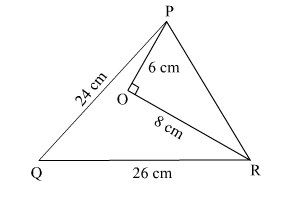# In the given figure, O is a point inside a ∆PQR such that ∠POR = 90°, OP = 6 cm and OR = 8 cm.Question:

In the given figure, O is a point inside a ∆PQR such that ∠POR = 90°, OP = 6 cm and OR = 8 cm. If PQ = 24 cm and QR = 26 cm, prove that ∆PQR is right-angled.Solution:

Applying Pythagoras theorem in right-angled triangle POR, we have:

$\mathrm{PR}^{2}=\mathrm{PO}^{2}+\mathrm{OR}^{2}$

$\Rightarrow \mathrm{PR}^{2}=6^{2}+8^{2}=36+64=100$

$\Rightarrow \mathrm{PR}=\sqrt{100}=10 \mathrm{~cm}$

In ∆ PQR,

$\mathrm{PQ}^{2}+\mathrm{PR}^{2}=24^{2}+10^{2}=576+100=676$

and $\mathrm{QR}^{2}=26^{2}=676$

$\therefore \mathrm{PQ}^{2}+\mathrm{PR}^{2}=\mathrm{QR}^{2}$

Therefore, by applying Pythagoras theorem, we can say that ∆PQR is right-angled at P.